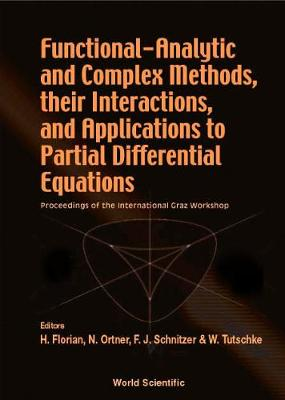•Functional-analytic And Complex Methods, Their Interactions, And Applications To Partial Differential Equations - Proceedings Of The International Graz Workshop (Hardback)

(editor), (editor), (editor), (editor)
£180.00
Hardback 472 Pages / Published: 12/11/2001
• We can order this

Usually dispatched within 3 weeks

Functional analysis is not only a tool for unifying mathematical analysis, but it also provides the background for today's rapid development of the theory of partial differential equations. Using concepts of functional analysis, the field of complex analysis has developed methods (such as the theory of generalized analytic functions) for solving very general classes of partial differential equations.This book is aimed at promoting further interactions of functional analysis, partial differential equations, and complex analysis including its generalizations such as Clifford analysis. New interesting problems in the field of partial differential equations concern, for instance, the Dirichlet problem for hyperbolic equations. Applications to mathematical physics address mainly Maxwell's equations, crystal optics, dynamical problems for cusped bars, and conservation laws. remove remove

Publisher: World Scientific Publishing Co Pte Ltd
ISBN: 9789810247645
Number of pages: 472
Weight: 726 g
Dimensions: 230 x 162 x 29 mm